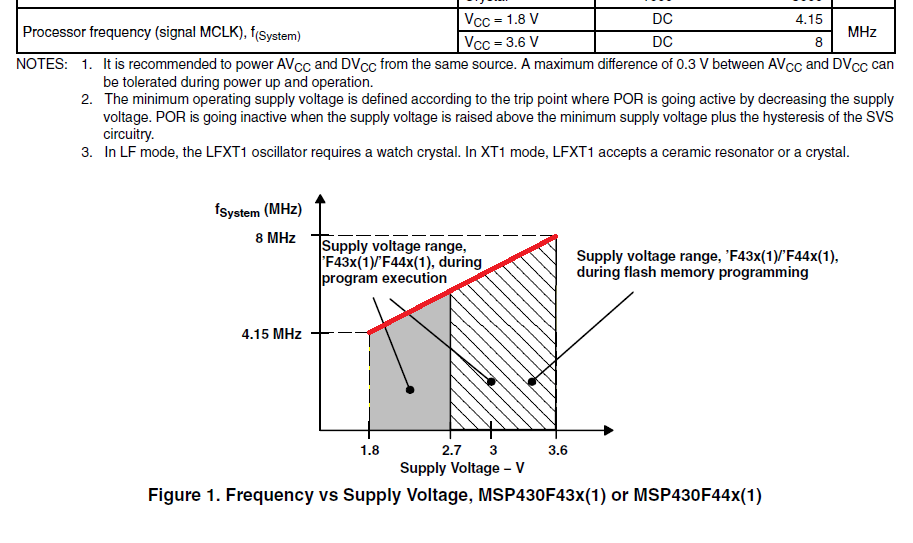If you have a related question, please click the "Ask a related question" button in the top right corner. The newly created question will be automatically linked to this question.

# MSP430F449: Linearity of Processor frequency f(System) to Supply Voltage

Part Number: MSP430F449

Hello,

The DS page-37 tells the recommended operating condition for Processor frequency (signal MCLK) f(System). There are a table and the Figure 1.

The Figure 1 has a line between the two points:

(Supply Voltage, fSystem ) = (1.8V, 4.15MHz) and (3.6V, 8MHz).

Then, is it possible to expect the line between the two points are Linear or not ?

As the background, my customer asked me if the CPU works at 8MHz under Vcc=3.3V. We would like to decide the CPU clock frequency under the supply voltage which is not specified.• Hello,

many thanks for contacting us on this topic. Yes, the characteristic can be assumed as linear.

This means, translating the graph into a slope of the characteristic results in 2.138MHz/V slope. This means e.g. at 2.7V the specified maximum operating frequency would be fmax(2.7V) = 8MHz - (0.9V * 2.138MHz/V) = 6.075MHz.